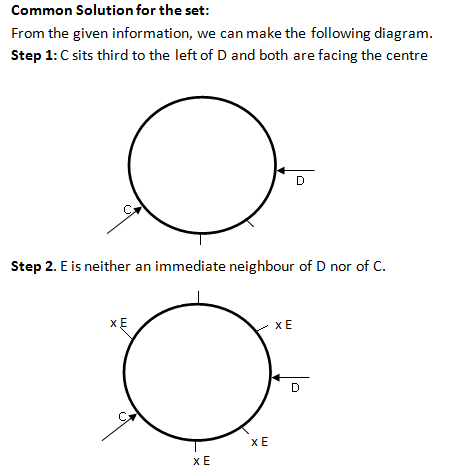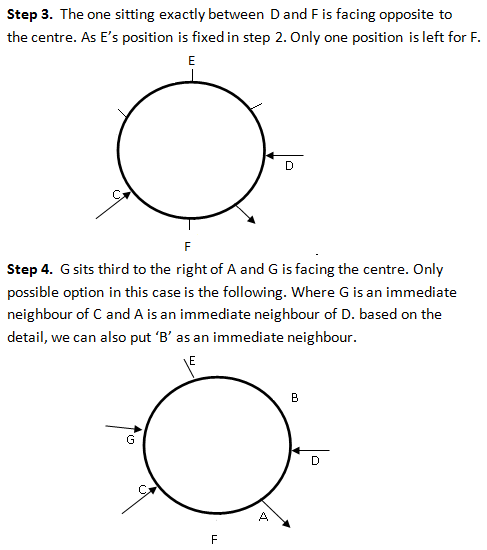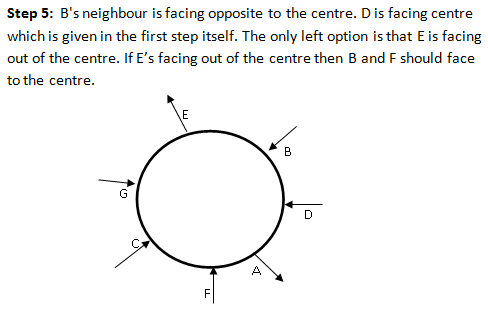### Logical Reasoning questions: Logical Reasoning questions that give you good practice for exams

Logical Reasoning questions are based on a problem set that give you good practice of direction and position concepts. Solve Logical Reasoning questions to test your understanding on arrangement concepts and later refer the solutions provided to tally your answers and to learn if there is any better approach. Trying to solve Logical Reasoning questions by timing yourself can help improve your score.

Directions for the question set:
Study the following information and answer the questions given :
Seven people A, B, C, D, E, F and G are sitting in a circle. Five of them are facing the centre while two of them are facing opposite to the centre. C sits third to the left of D and both are facing the centre. E is neither an immediate neighbour of D nor of C. The one sitting exactly between D and F is facing opposite to the centre. G sits third to the right of A and G is facing the centre. One of B’s neighbour is facing opposite to the centre.

1. Which of the following pairs represents persons, facing opposite to the centre?
(a) A and F
(b) E and F
(c) A and E
(d) Cannot be determined
(e) None of these

2. Who is sitting second to the left of A?
(a) C
(b) G
(c) E
(d) B
(e) None of these

3. Who is sitting to the immediate left of E?
(a) C
(b) G
(c) B
(d) A
(e) None of these

4. What is the position of F with respect to B?
(a) Fourth to the left
(b) Second to the right
(c) Third to the right
(d) Second to the left
(e) None of these

5. If all the persons are asked to sit in a clockwise direction in an alphabetical order starting from A, the position of how many will remain unchanged, excluding A?
(a) Three
(b) One
(c) Two
(d) None
(e) Four

### Answers and Explanations: Click the down arrow to expandAnswer 1: (c) A and E are the pair facing opposite to the centre. Correct option is (c)

Answer 2: (d) B sits second to the left of A. Correct option is (d)

Answer 3: (b) G is sitting to the immediate left of E. Correct option is (b)

Answer 4: (e) F is third to the left of B. Correct option is (e).

Answer 5: (c) The positions of C and E remain unchanged except A. Correct option is (c).

Extra tips for Logical Reasoning questions:
• Whenever you begin to read Logical Reasoning questions, start decoding the information from the very start as when you read ahead, you can clearly understand the relationship between objects/people.
• Logical Reasoning questions may look lengthy. But when you read and use the diagram approach to solve them, they may become easier than you think they are.
• Practice Logical Reasoning questions from various topics to get a hold on all the commonly tested concepts.